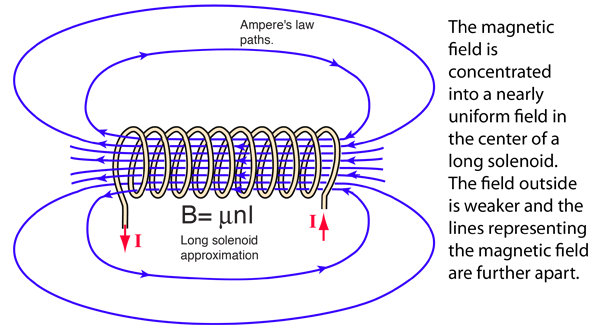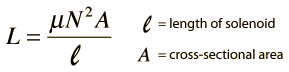Inductance of a SolenoidThe inductance of a coil of wire is given bySolenoid length cm with N = turns,

Coil radius r = cm gives area A = cm2.

Relative permeability of the core k = ,

Then the inductance of the solenoid is

L = Henry = mH.

This is a single purpose calculation which gives you the inductance value when you make any change in the parameters.

Small inductors for electronics use may be made with air cores. For larger values of inductance and for transformers, iron is used as a core material. The relative permeability of magnetic iron is around 200.

This calculation makes use of the long solenoid approximation. It will not give good values for small air-core coils, since they are not good approximations to a long solenoid.

 Inductance of a toroid
Index

Inductance concepts

AC Circuits

 HyperPhysics***** Electricity and Magnetism R Nave
Go Back